Learn to Code via Tutorials on Repl.it!

← Back to all posts
How an ALU works.

Hey.

I hope you are well.

Let's start.

# What is an ALU?

The ALU, or arithmetic and logic unit, is a component inside your computer that performs, well, arithmetic and logic.

It used to be it's own chip but now is embedded in your CPU.

To understand the ALU is to understand logic gates so we will go in-depth on those as well.

# Logic gates.

Think of binary.

It uses 1s and 0s right?

So two values.

• 1
• 0

Think of something that also uses two values.

Bools!

• True
• False

And we need 1 more thing to complete this logic.

Electricity!

The absence of electricity flowing through a wire is represented as a 0 and an electric current is represented as a 1.

• On
• Off

So we can map it all up like this:

#### The chart:

• Bools
• True
• False
• Binary
• 1
• 0
• Electricty
• On
• Off

Okay!

So now that we have that established how do we make logic gates?

Well, we can connect certain transistors in a way that it creates these circuits.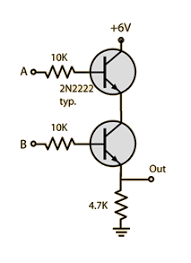The main gates we use are the following:

• AND
• OR
• NOT
• XOR

Let's discuss these in detail.

## AND gates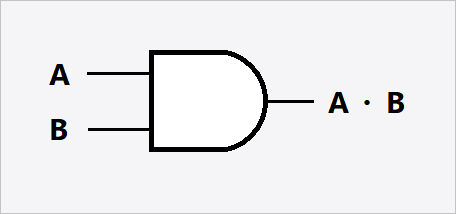Above is the symbolic representation of an AND gate. We abstract away all the wires, and use this symbol.

The typical AND gate takes 2 inputs (A and B) and returns 1 output. This output (if we represented electricity as binary) is A * B.

Note that if we added, for example C, it would be the same output but it would be A B C.

## OR gates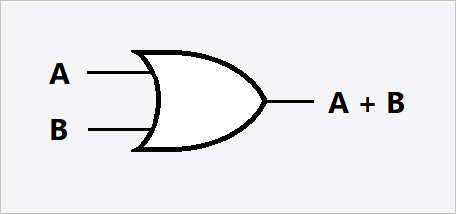The typical OR gate takes 2 inputs (A and B) and returns A + B.

But wait, you might be saying, binary has no representation of 2! Well if it has a 2 it just makes it a 1.

Again, note that if we added, for example C, it would be the same output but it would be A + B + C

## NOT gates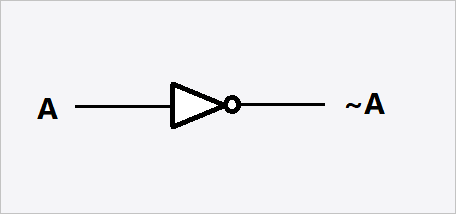The NOT gate takes 1 input and returns the opposite of it.

Example:

• 1 turns into a 0
• 0 turns into a 1

## XOR gatesThe XOR gate is very similar to the OR gate.

If only one of the inputs is 1 it returns a 1.

Again, note that if we added, for example C, it would be the same output just with 3 inputs and only 1 could be on.

# How is this relevant to the ALU?

The ALU uses these gates to make circuits.

For example: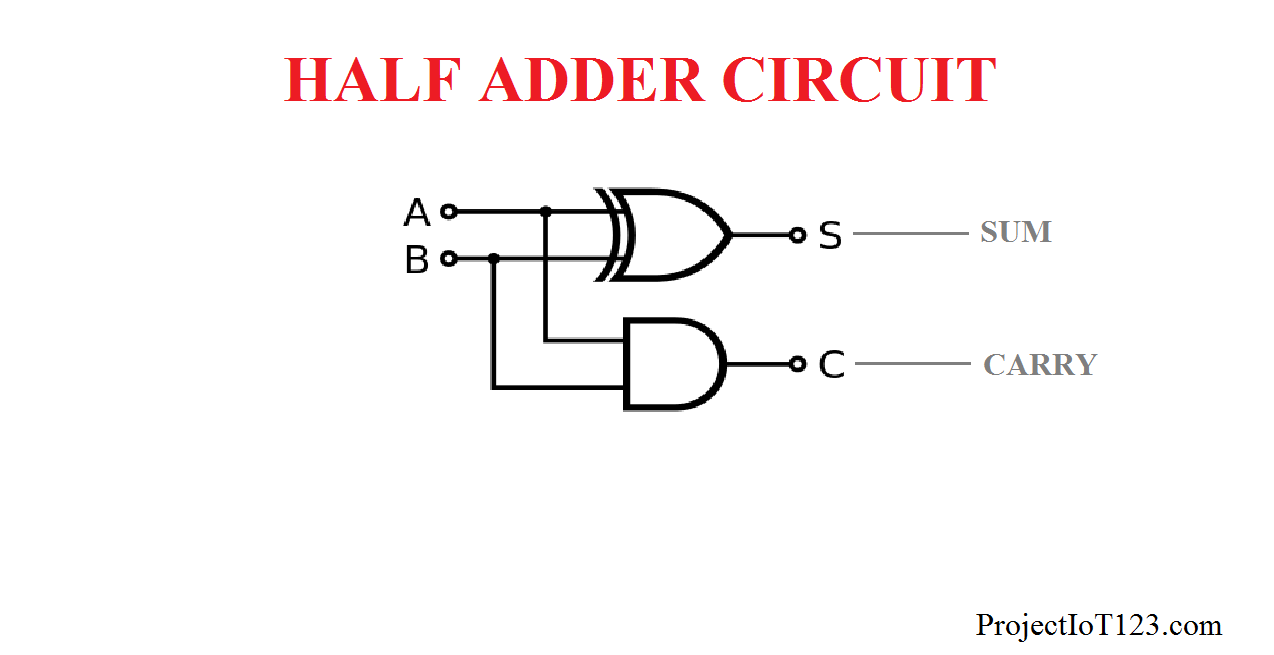This is a half adder. It takes two inputs (A and B) and combines them. If there is a carry into the next bit though. It will send a 1 on the carry line.

This can be used to make this circuit: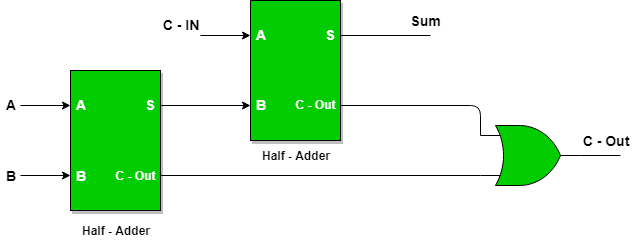Which is a full adder. It s the same as the half adder but takes 3 inputs.

And we can use that to make this: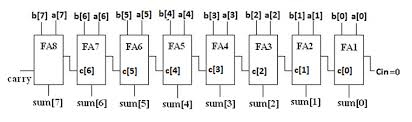It is an 8-bit ripple-carry adder. It can add 8 bit numbers.

# Conclusion

If you enjoyed, upvote.

And the ALU is very complex but very cool.

AtriDey (171)

An ALU may be complex, but an allu is a Finnish guy who shoots you in the head.

RolandJLevy (753)

Hi @Wuru, thanks so much for the helpful and concise explanation in plain English - excellent 😀

JustAWalrus (1167)

Thank you, it is kind people like you that keep me motivated to create these tutorials. @rjlevy

RolandJLevy (753)

@Wuru thanks - I can see you put a lot of effort into it. It was very easy to follow. What other tutorials have you created?

RolandJLevy (753)

Thanks @Wuru - I'll check them out. You don't often see tutorials on repl.it